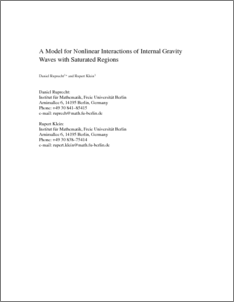Repository: Freie Universität Berlin, Math Department

# A Model for Nonlinear Interactions of Internal Gravity Waves with Saturated Regions

Ruprecht, D. and Klein, R. (2011) A Model for Nonlinear Interactions of Internal Gravity Waves with Saturated Regions. Meteorologische Zeitschrift, 20 (2). pp. 243-252.Preview

195kB

## Abstract

A model for interactions between non-hydrostatic gravity waves and deep convective narrow hot towers is presented. The starting point of the derivation are the conservation laws for mass, momentum and energy for compressible flows combined with a bulk micro-physic model. Using multiscale asymptotics, a set of leading order equations is extracted, valid for the specific scales of the investigated regime. These are a timescale of 100 s, a horizontal and vertical lengthscale of 10 km for the wave dynamics plus a second horizontal lengthscale of 1 km for the narrow hot towers. Because of the comparatively short horizontal scales, Coriolis effects are negligible in this regime. The leading order equations are then closed by applying conditional averages over the hot tower lengthscale, leading to a closed model for the wave-scale that retains the net effects of the smaller scale dynamics. By assuming a systematically small saturation deficit in the ansatz, the small vertical displacements arising in this regime suffice to induce leading order changes of the saturated area fraction. The latter is the essential parameter in the model arising from the micro-physics.

Item Type: Article Mathematical and Computer Sciences > MathematicsMathematical and Computer Sciences > Mathematics > Applied Mathematics Department of Mathematics and Computer Science > Institute of Mathematics > Geophysical Fluid Dynamics Group 918 Ulrike Eickers 26 Jul 2010 12:41 03 Mar 2017 14:40

Repository Staff Only: item control page